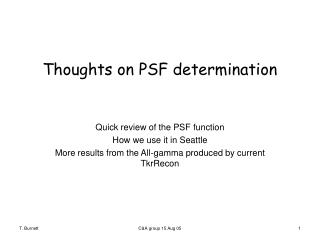DownloadDownload PresentationThoughts on PSF determination

# Thoughts on PSF determination

Télécharger la présentation## Thoughts on PSF determination

- - - - - - - - - - - - - - - - - - - - - - - - - - - E N D - - - - - - - - - - - - - - - - - - - - - - - - - - -
##### Presentation Transcript

1. Thoughts on PSF determination Quick review of the PSF function How we use it in Seattle More results from the All-gamma produced by current TkrRecon C&A group 15 Aug 05

2. The PSF function • See the discussion in the LATdoc • Define  to be the deviation between actual and reconstructed directions. • Assume (for now!) that there is no azimuthal dependence about the actual direction. The normalized function, expressed as a differential in  (and assuming small angles) is:where the only two parameters are a scale factor  and the power law  • Interesting cases are the  limit, corresponding exactly to the product of Gaussians in the two projections with standard deviation , and =2, the Breit-Wigner case, below which the rms diverges. • Note that it only depends on 2, and is simpler to use as a distribution in the square of the deviation. In the LAT doc we define u=0.5(/ ) 2 C&A group 15 Aug 05

3. The Seattle fit procedure • Bin the all-gamma data in 8 bins in cos (cos  =0.1) and bins in log(E) such that  log(E) =10, starting at 16 MeV, ending at 16 GeV (6 bins) or 160 GeV (8 bins). An additional cos “bin” sums the first 6 bins (0 to 66 degrees). • For each bin, make a histogram of x=log10(/S(E,cos ))), where S is a scaling function meant to minimize the binning effect, so that the  fit parameter will be approximately constant, and, by iteration, close to one over the range of the bin. • Fit each histogram to the function Note that this separates the scale and the shape variables, and makes no assumptions about how they are related. (The assertion to the contrary last week is not correct.) The actual ROOT fit function is show below. P is the (redundant?) normalization, and p and p are the parameters for the scaled sigma and gamma. Also see the scale factor function. double PointSpreadFunction::scaleFactor(double energy,double zdir, bool thin) { // following numbers determined empirically to roughly // give a fit scale factor of 1.0 independent of energy double t = pow( energy/100., -0.84); double zfactor = 1.0 + 0.54*(1-fabs(zdir)); // from trendline fits to log10 binned results double x = 2.0*log10(energy/100.)+2.0; double efactor=1.0; if( thin ){ efactor*= (0.0205*x*x -0.148*x +1.1132); return efactor*zfactor*sqrt( sqr(27e-3*t) + sqr( 225E-6) ); }else{ efactor*= (-0.064*x + 1.0757); return efactor*zfactor*sqrt( sqr(44e-3*t) + sqr( 358e-6) ); } } inline double sqr(double x){return x*x;} double psf_function( double * x, double * p) { double sigma = p, gamma = p; double qsq = ::pow(10., 2* (*x))/2/sqr(sigma); return p * (1-1/gamma) * pow( 1.+qsq/gamma ,-gamma); } C&A group 15 Aug 05

4. Example fit “Corner”: define the scale parameter  Density at the source Power law: the tail parameter  Solid angle density (arbitrary units) C&A group 15 Aug 05

5. Events/run satisfying cuts Run number The data: described last week • 500 runs of 10K all_gamma: 5 M generated Note: this allows an easy calculation of the effective area C&A group 15 Aug 05

6. Question: can one define a simple scale function of energy and incident angle for which the fit values of ’ and  are ~ constant?? C&A group 15 Aug 05

7. Remaining sigma energy and angle dependence C&A group 15 Aug 05

8. The  energy dependence C&A group 15 Aug 05

9. Gamma angular dependence C&A group 15 Aug 05